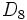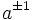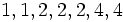# Dihedral group:D16

View a complete list of particular groups (this is a very huge list!)[SHOW MORE]

## Definition

### Definition by presentation

The dihedral group$\! D_{16}$, sometimes denoted$D_8$, also called the dihedral group of order sixteen or the dihedral group of degree eight or the dihedral group acting on eight elements, is a dihedral group defined by the presentation:$\langle a,x \mid a^8 = x^2 = e, xax^{-1} = a^{-1} \rangle$.

where$e$ is the identity element.

The element$a$ is termed a rotation or a generator of the cyclic piece and the element$x$ is termed a reflection.

Note that the notation$\! D_8$ is more commonly used to denote the dihedral group of order eight.

### Geometric definition

The dihedral group$D_{16}$ (also called$D_8$) is defined as the group of all symmetries of the regular octagon. This has a cyclic subgroup comprising rotations (which is the cyclic subgroup generated by$a$) and has eight reflections each being an involution: four reflections about lines joining midpoints of opposite sides, and four reflections about diagonals.

### Definition as a permutation group

The group can be defined as the subgroup of the symmetric group on$\{ 1,2,3,4,5,6,7,8 \}$ generated by a cycle of length$8$ and a reflection:$\! \langle (1,2,3,4,5,6,7,8), (1,8)(2,7)(3,6)(4,5) \rangle$

## Elements

Further information: element structure of dihedral group:D16

### Upto conjugacy

There are seven conjugacy classes of elements:

1. The identity element. (1)
2. The rotation by$\pi$, which is given by$a^4$ in the presentation. (1)
3. The rotations by$\pm \pi/2$, which are given by$a^{\pm 2}$ in the presentation. (2)
4. The rotations by$\pm \pi/4$, which are given by$a^{\pm 1}$ in the presentation. (2)
5. The rotations by$\pm 3\pi/4$, which are given by$a^{\pm 3}$ in the presentation. (2)
6. The rotations about axes joining opposite vertices, given by$x, a^2x, a^4x, a^6x$ in the presentation. (4)
7. The rotations about axes joining midpoints of opposite sides, given by$ax, a^3x, a^5x, a^7x$ in the presentation. (4)

### Upto automorphism

Under the action of outer automorphisms, the conjugacy classes (4) and (5) combine, and the conjugacy classes (6) and (7) combine. Thus, there are five equivalence classes of sizes$1,1,2,4,8$.

## Arithmetic functions

### Basic arithmetic functions

Want to compare and contrast arithmetic function values with other groups of the same order? Check out groups of order 16#Arithmetic functions
Function Value Similar groups Explanation for function value GAP verification (set G := DihedralGroup(16);) -- See more at #GAP implementation
underlying prime of p-group 2
order (number of elements, equivalently, cardinality or size of underlying set) 16 groups with same order Order(G); using Order.
prime-base logarithm of order 4 groups with same prime-base logarithm of order
max-length of a group 4 max-length of a group equals prime-base logarithm of order for group of prime power order
chief length 4 chief length equals prime-base logarithm of order for group of prime power order
composition length 4 composition length equals prime-base logarithm of order for group of prime power order
exponent of a group 8 groups with same order and exponent of a group | groups with same exponent of a group cyclic subgroup of order 8. Exponent(G); using Exponent.
prime-base logarithm of exponent 3 groups with same order and prime-base logarithm of exponent | groups with same prime-base logarithm of order and prime-base logarithm of exponent | groups with same prime-base logarithm of exponent
nilpotency class 3 groups with same order and nilpotency class | groups with same prime-base logarithm of order and nilpotency class | groups with same nilpotency class NilpotencyClassOfGroup(G); using NilpotencyClassOfGroup.
derived length 2 groups with same order and derived length | groups with same prime-base logarithm of order and derived length | groups with same derived length the derived subgroup is contained in the cyclic subgroup and is hence abelian DerivedLength(G); using DerivedLength.
Frattini length 3 groups with same order and Frattini length | groups with same prime-base logarithm of order and Frattini length | groups with same Frattini length The first Frattini subgroup is cyclic generated by$a^2$, each subsequent Frattini subgroup moves to the set of squares of its predecessor. FrattiniLength(G); using FrattiniLength.
minimum size of generating set 2 groups with same order and minimum size of generating set | groups with same prime-base logarithm of order and minimum size of generating set | groups with same minimum size of generating set Rank(G); using Rank.
subgroup rank of a group 2 groups with same order and subgroup rank of a group | groups with same prime-base logarithm of order and subgroup rank of a group | groups with same subgroup rank of a group All proper subgroups are cyclic, dihedral, or Klein four-groups. SubgroupRank(G); using SubgroupRank.
rank of a p-group 2 groups with same order and rank of a p-group | groups with same prime-base logarithm of order and rank of a p-group | groups with same rank of a p-group there exist Klein four-subgroups. RankAsPGroup(G); using RankAsPGroup.
normal rank of a p-group 1 groups with same order and normal rank of a p-group | groups with same prime-base logarithm of order and normal rank of a p-group | groups with same normal rank of a p-group all abelian normal subgroups are cyclic. NormalRank(G); using NormalRank.
characteristic rank of a p-group 1 groups with same order and characteristic rank of a p-group | groups with same prime-base logarithm of order and characteristic rank of a p-group | groups with same characteristic rank of a p-group All abelian characteristic subgroups are cyclic. CharacteristicRank(G); using CharacteristicRank.

### Arithmetic functions of a counting nature

Function Value Similar groups Explanation
number of conjugacy classes 7 groups with same order and number of conjugacy classes | groups with same prime-base logarithm of order and number of conjugacy classes | groups with same number of conjugacy classes As$D_{2n}, n = 8$,$n$ even:$(n + 6)/2 = (8 + 6)/2 = 7$
number of equivalence classes under rational conjugacy 6 groups with same order and number of equivalence classes under rational conjugacy | groups with same prime-base logarithm of order and number of equivalence classes under rational conjugacy | groups with same number of equivalence classes under rational conjugacy
number of conjugacy classes of rational elements 5 groups with same order and number of conjugacy classes of rational elements | groups with same prime-base logarithm of order and number of conjugacy classes of rational elements | groups with same number of conjugacy classes of rational elements

### Lists of numerical invariants

List Value Explanation/comment
conjugacy class sizes$1,1,2,2,2,4,4$ See element structure of dihedral group:D16 and element structure of dihedral groups
order statistics$1 \mapsto 1, 2 \mapsto 9, 4 \mapsto 2, 8 \mapsto 4$ See element structure of dihedral group:D16 and element structure of dihedral groups
orders of subgroups$1$,$2$ (9 times),$4,4,4,4,4,8,8,8,16$ See subgroup structure of dihedral group:D16 and subgroup structure of dihedral groups
degrees of irreducible representations$1,1,1,1,2,2,2$ See linear representation theory of dihedral group:D16 and linear representation theory of dihedral groups

## Group properties

Property Satisfied? Explanation Comment
group of prime power order Yes
nilpotent group Yes prime power order implies nilpotent
supersolvable group Yes via nilpotent: finite nilpotent implies supersolvable
solvable group Yes via nilpotent: nilpotent implies solvable
Abelian group No$a$ and$x$ don't commute
Metacyclic group Yes Cyclic normal subgroup of order eight, cyclic quotient of order two
T-group No$\langle a^4,x \rangle \triangleleft \langle a^2,x \rangle$, which is normal, but$\langle a^4, x \rangle$ is not normal
Monolithic group Yes Unique minimal normal subgroup of order two
One-headed group No Three distinct maximal normal subgroups of order eight
SC-group No
ACIC-group Yes Every automorph-conjugate subgroup is characteristic
Rational group No The elements$a, a^3$ generate the same cyclic group but are not conjugate Smallest order for a non-abelian$2$-group that is not rational.
Rational-representation group No The representation as symmetries of a regular octagon is not rational
Ambivalent group Yes dihedral groups are ambivalent Also see generalized dihedral groups are ambivalent.
Directly indecomposable group Yes
Centrally indecomposable group Yes
Splitting-simple group No

## Elements

Further information: element structure of dihedral group:D16

## Subgroups

Further information: subgroup structure of dihedral group:D16

In case a single equivalence class of subgroups under automorphisms comprises multiple conjugacy classes of subgroups, outer curly braces are used to bucket the conjugacy classes.

Automorphism class of subgroups List of subgroups Isomorphism class Order of subgroups Index of subgroups Number of conjugacy classes (=1 iff automorph-conjugate subgroup) Size of each conjugacy class (=1 iff normal subgroup) Total number of subgroups (=1 iff characteristic subgroup) Isomorphism class of quotient (if exists) Subnormal depth Nilpotency class
trivial subgroup$\{ e \}$ trivial group 1 16 1 1 1 dihedral group:D16 1 0
center of dihedral group:D16$\{ e, a^4 \}$ cyclic group:Z2 2 8 1 1 1 dihedral group:D8 1 1
non-normal order two subgroups of dihedral group:D16$\{ \{ e,x \}, \{ e, a^2x \}, \{ e, a^4x \}, \{ e, a^6x \} \}$$\{ \{ e, ax \}, \{ e, a^3x \}, \{ e, a^5x \}, \{ e, a^7x \} \}$
cyclic group:Z2 2 8 2 4 8 -- 3 1
derived subgroup of dihedral group:D16$\{ e, a^2, a^4, a^6 \}$ cyclic group:Z4 4 4 1 1 1 Klein four-group 1 1
Klein four-subgroups of dihedral group:D16$\{ \{ e, a^4, x, a^4x \}, \{ e, a^4, a^2x, a^6x \} \}$$\{ \{ e, a^4, ax, a^5x \}, \{ e, a^4, a^3x, a^7x \} \}$
Klein four-group 4 4 2 2 4 -- 2 1
Cyclic maximal subgroup of dihedral group:D16$\{ e, a, a^2, a^3, a^4, a^5, a^6, a^7 \}$ cyclic group:Z8 8 2 1 1 1 cyclic group:Z2 1 1
D8 in D16$\langle a^2, x \rangle$,$\langle a^2, ax \rangle$ dihedral group:D8 8 2 2 1 2 cyclic group:Z2 1 2
whole group all elements dihedral group:D16 16 1 1 1 1 trivial group 0 3
Total (8 rows) -- -- -- -- 11 -- 19 -- -- --

## GAP implementation

### Group ID

This finite group has order 16 and has ID 7 among the groups of order 16 in GAP's SmallGroup library. For context, there are 14 groups of order 16. It can thus be defined using GAP's SmallGroup function as:

SmallGroup(16,7)

For instance, we can use the following assignment in GAP to create the group and name it$G$:

gap> G := SmallGroup(16,7);

Conversely, to check whether a given group$G$ is in fact the group we want, we can use GAP's IdGroup function:

IdGroup(G) = [16,7]

or just do:

IdGroup(G)

to have GAP output the group ID, that we can then compare to what we want.

### Hall-Senior number

This group of prime power order has order 16 and has Hall-Senior number 12 among the groups of order 16. This information can be used to construct the group in GAP using the Gap3CatalogueGroup function as follows:

Gap3CatalogueGroup(16,12)

WARNING: There is some disagreement between the GAP 3 catalogue numbers and the Hall-Senior numbers for some abelian groups, but it does not affect this group.

For instance, we can use the following assignment in GAP to create the group and name it$G$:

gap> G := Gap3CatalogueGroup(16,12);

Conversely, to check whether a given group$G$ is in fact the group we want, we can use GAP's Gap3CatalogueIdGroup function:

Gap3CatalogueIdGroup(G) = [16,12]

or just do:

Gap3CatalogueIdGroup(G)

to have GAP output the group ID, that we can then compare to what we want.

### Other descriptions

Description Functions used
DihedralGroup(16) DihedralGroup